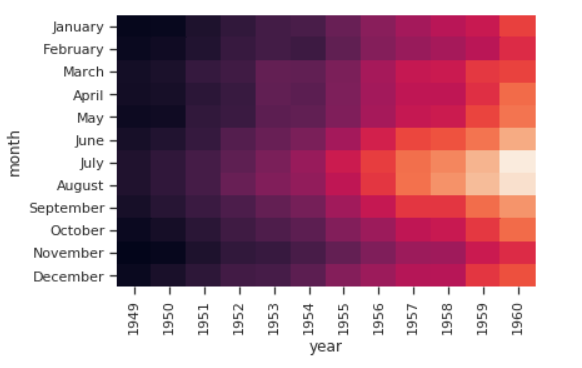# seaborn 高级绘图

## FacetGrid

FacetGrid 是 seaborn 库当中用来绘制数据集当中的某些子集的方法，利用该方法可以很轻松的绘制出数据集当中子集的情形。

``````%matplotlib inline
import numpy as np
import pandas as pd
import seaborn as sns
from scipy import stats
import matplotlib as mpl
import matplotlib.pyplot as plt

sns.set(style="ticks")
np.random.seed(sum(map(ord, "axis_grids")))
``````

``````tips = sns.load_dataset("tips")
tips.head()
``````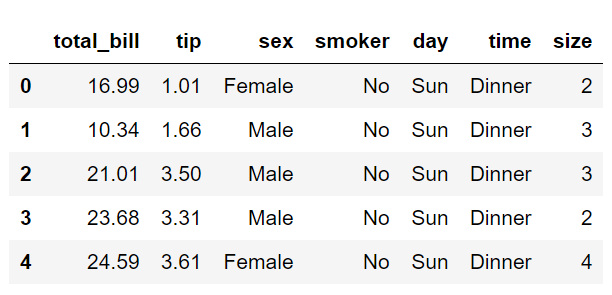``````g = sns.FacetGrid(tips, col="time")
``````
• tips：数据集
• col：想要表示的数据集的子集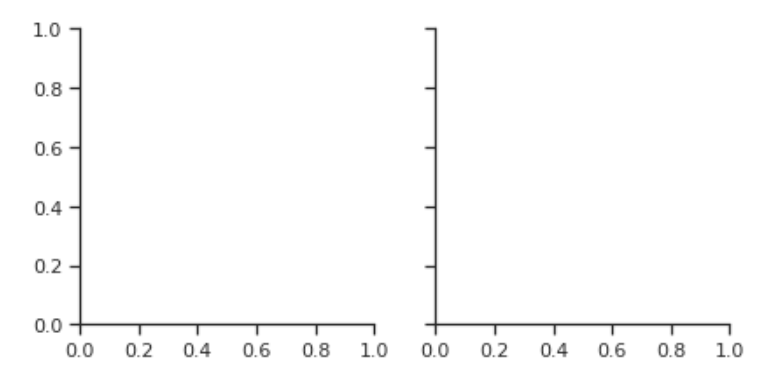### 直方图绘制

``````g = sns.FacetGrid(tips, col="time", size=4)
g.map(plt.hist, "tip")
``````

map 函数中所对应的属性：

• plt.hist：想要绘制的图形类型
• “tip”：x轴数据索引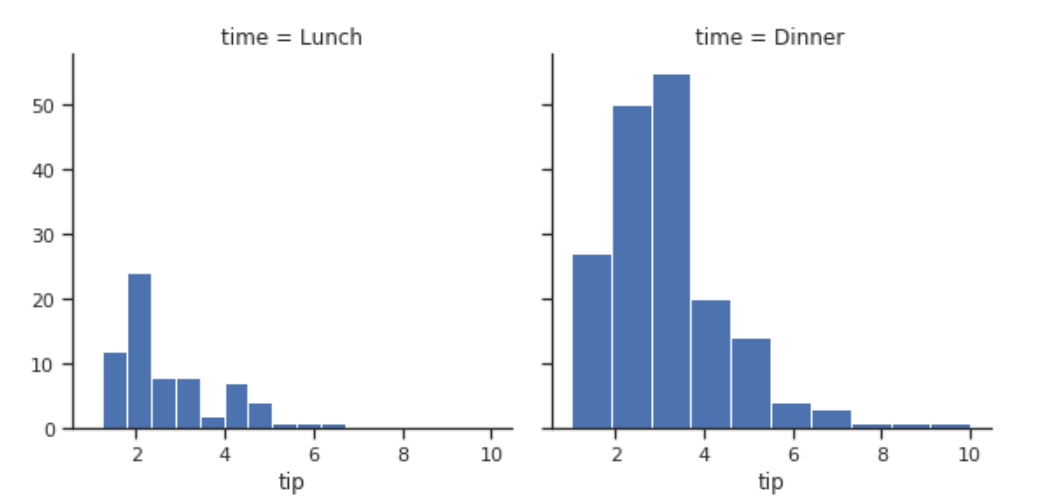### 散点图绘制

``````g = sns.FacetGrid(tips, col="sex", hue="smoker", size=4)
# alpha表示透明度
g.map(plt.scatter, "total_bill", "tip", alpha=0.7)
# 添加图示说明
g.add_legend()
``````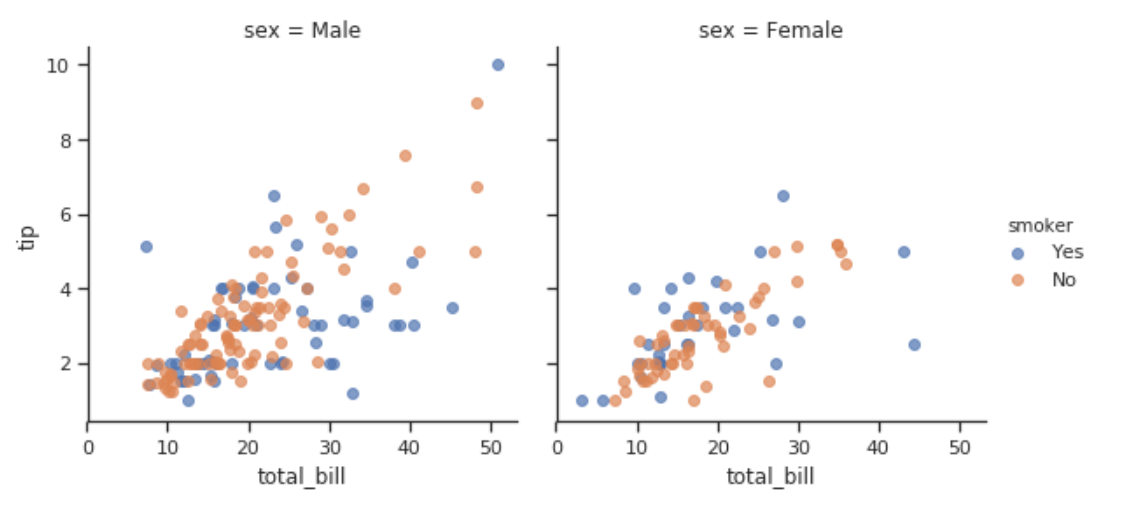``````# 指定现实的调色板
pal = dict(Lunch="seagreen", Dinner="gray")
g = sns.FacetGrid(tips, hue="time", palette=pal, size=6)
# s表示点的大小，linewidth线宽
g.map(plt.scatter, "total_bill", "tip", s=70, alpha=.7)
# 添加图示说明
g.add_legend();
``````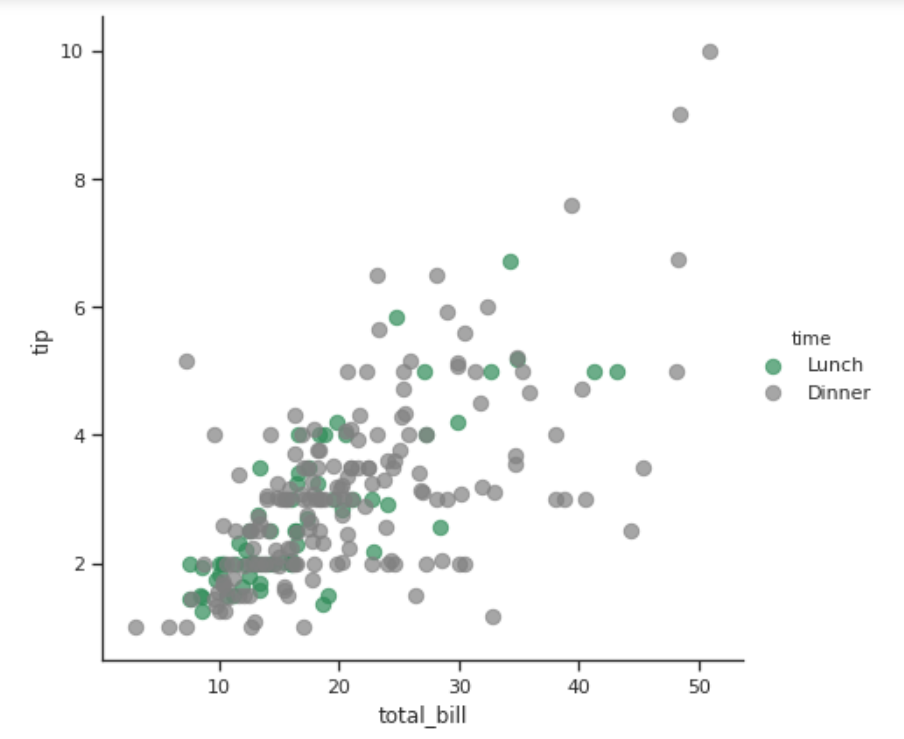``````g = sns.FacetGrid(tips, hue="sex", palette="Set1", size=5, hue_kws={"marker": ["^", "v"]})
g.map(plt.scatter, "total_bill", "tip", s=100, linewidth=.5, edgecolor="white")
g.add_legend();
``````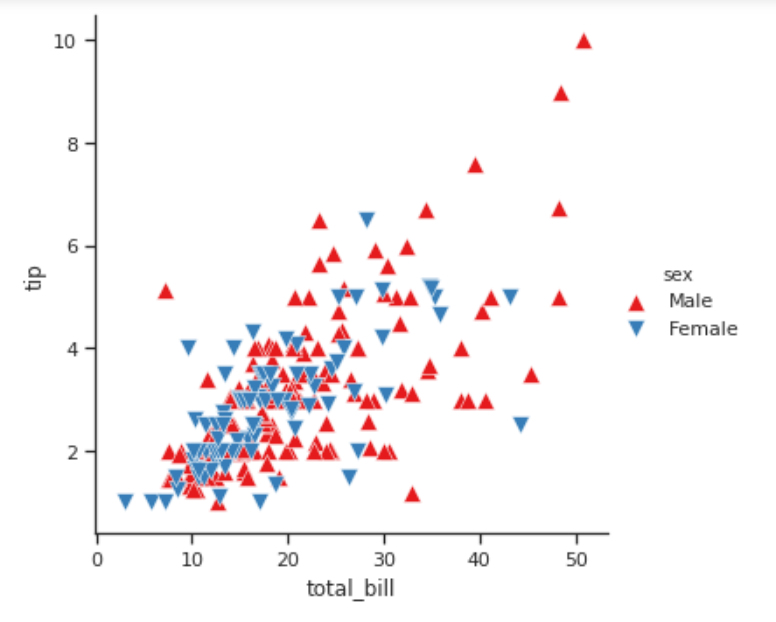``````g = sns.FacetGrid(tips, row="sex", col="smoker", margin_titles=True, size=2.5)

g.map(plt.scatter, "total_bill", "tip", color="#334488", edgecolor="white", lw=.5);
``````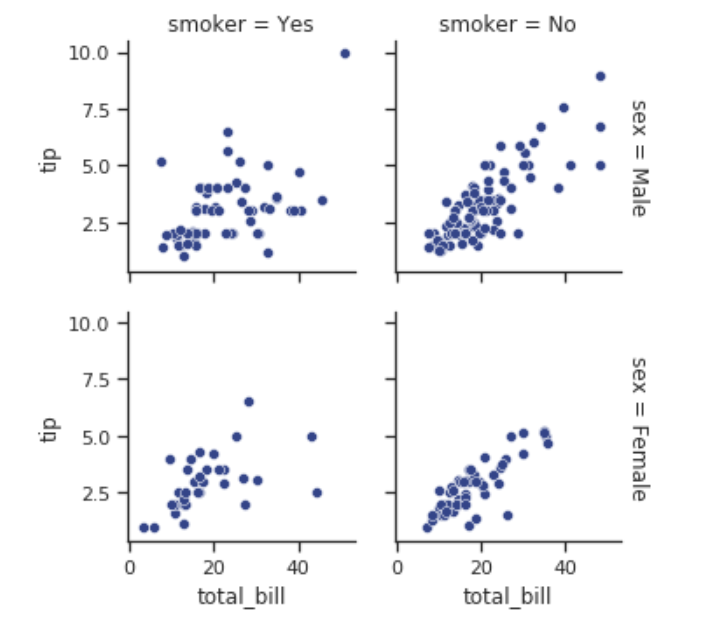### 回归图绘制

``````g = sns.FacetGrid(tips, col="time", row="smoker", margin_titles=True, size=4)
g.map(sns.regplot, "size", "total_bill", color=".1", fit_reg=False, x_jitter=.1)
``````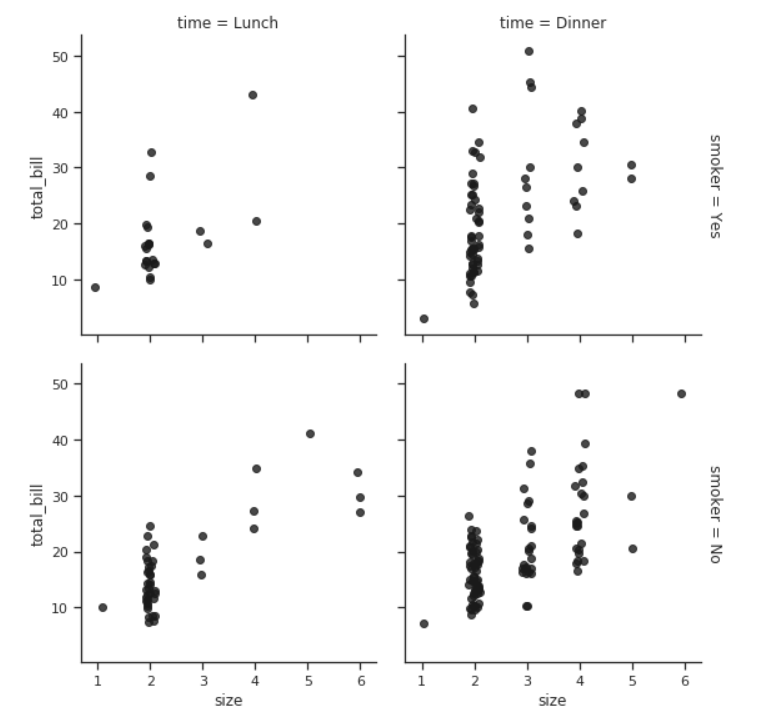### 柱状图绘制

``````g = sns.FacetGrid(tips, col="day", size=5, aspect=.5)
g.map(sns.barplot, "sex", "total_bill");
``````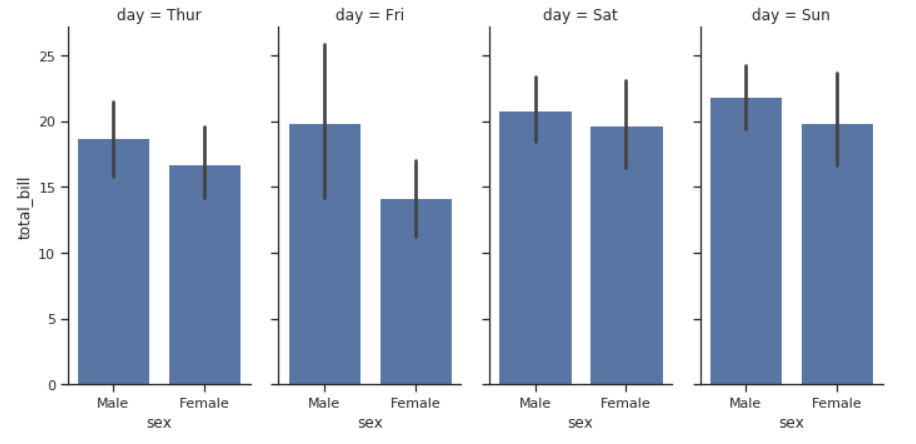### 柱状图绘制

``````g = sns.FacetGrid(tips, row="day",size=1.7, aspect=4)
g.map(sns.boxplot, "total_bill");
``````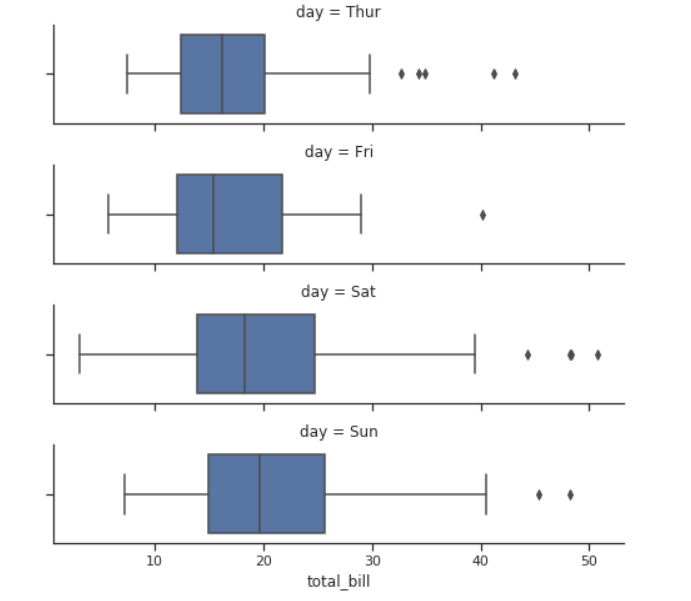## PairGrid

PairGrid 是将数据集中的成对属性进行组合展示

``````iris = sns.load_dataset("iris")
g = sns.PairGrid(iris)
g.map(plt.scatter);
``````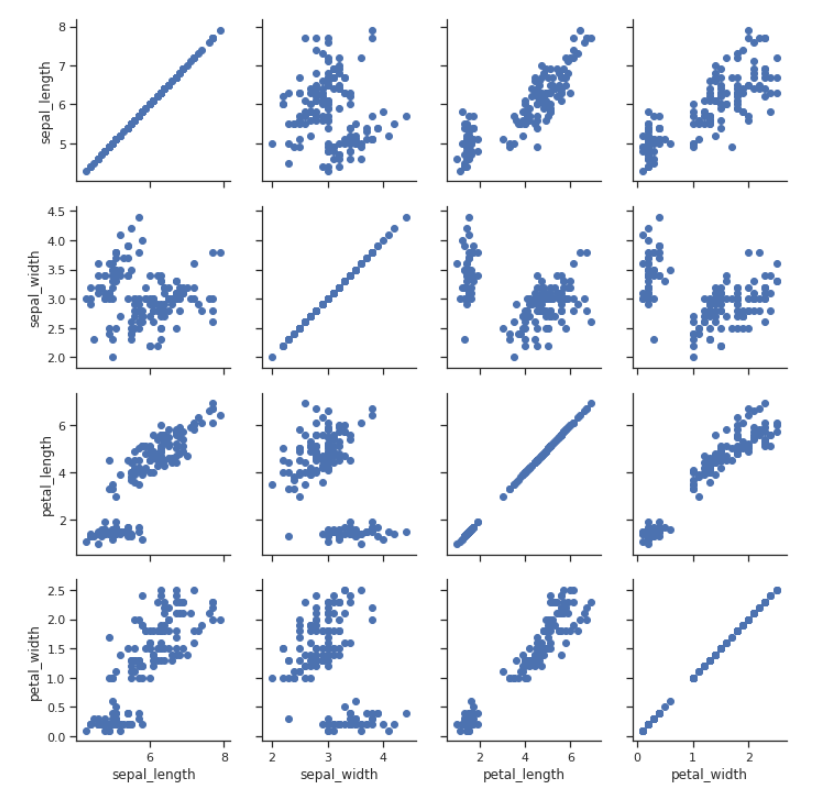``````g = sns.PairGrid(iris)
# 对角线显示的曲线样式
g.map_diag(plt.hist)
# 非对角线显示的曲线样式
g.map_offdiag(plt.scatter);
``````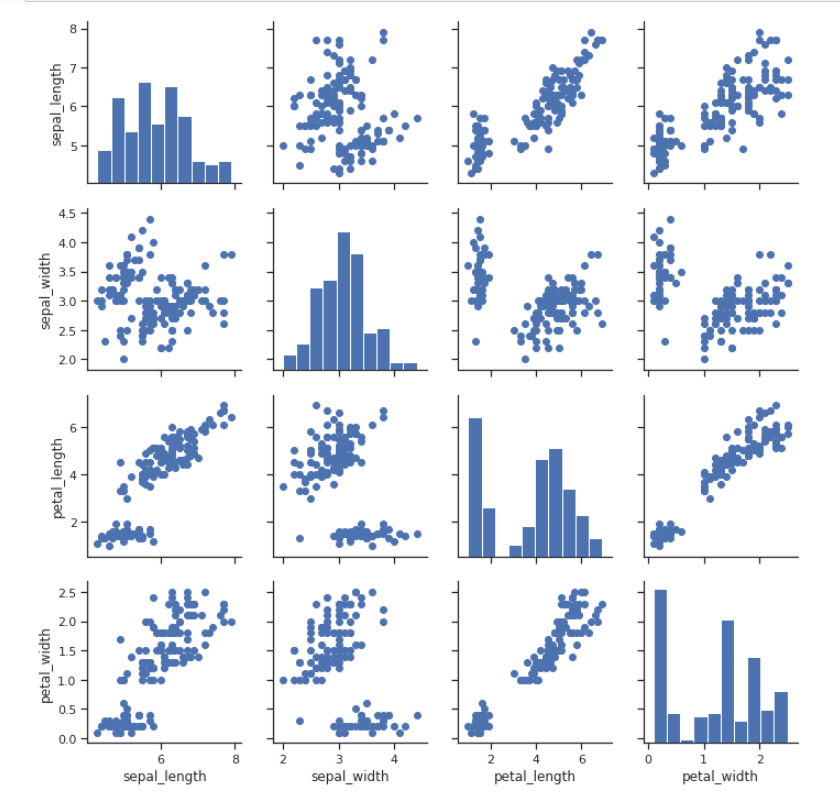``````g = sns.PairGrid(iris, hue="species")
g.map_diag(plt.hist)
g.map_offdiag(plt.scatter)
g.add_legend();
``````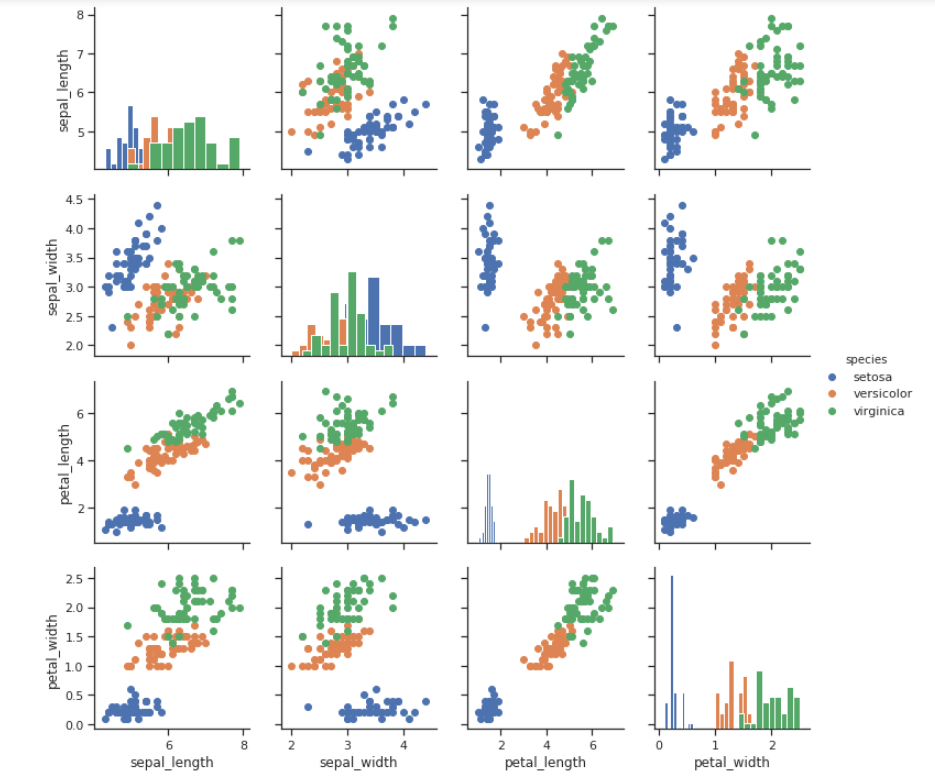``````g = sns.PairGrid(tips, hue="size", palette="GnBu_d", size=4)
g.map(plt.scatter, s=70, edgecolor="white")
g.add_legend();
``````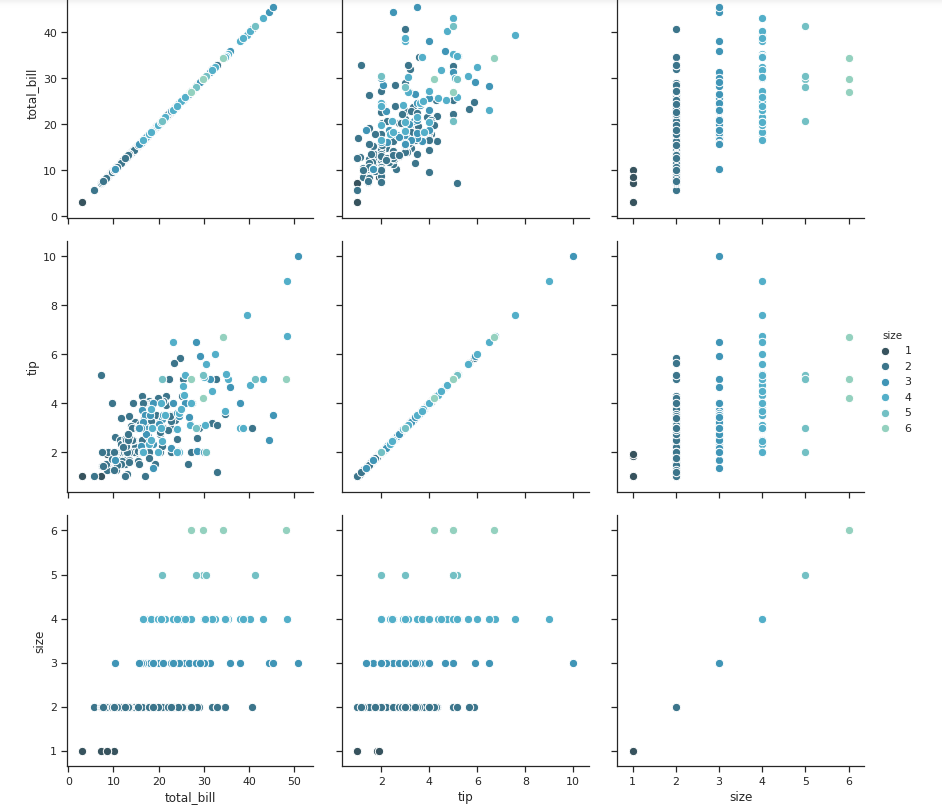## heatmap

heatmap 是热力图，可以用来显示数据的集中分布区域。

``````flights = sns.load_dataset("flights")
flights.head()
``````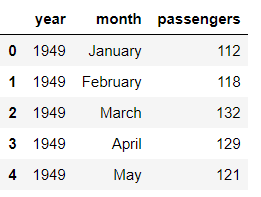``````flights = flights.pivot("month", "year", "passengers")
print (flights)
``````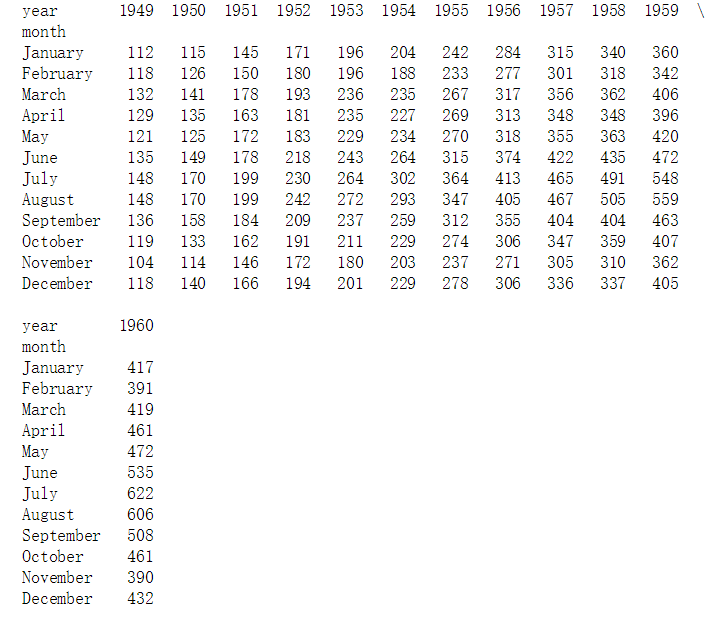``````ax = sns.heatmap(flights)
``````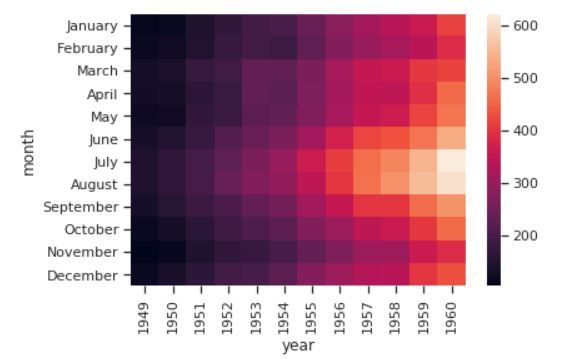### 填入具体数字

``````ax = sns.heatmap(flights, annot=True,fmt="d")
``````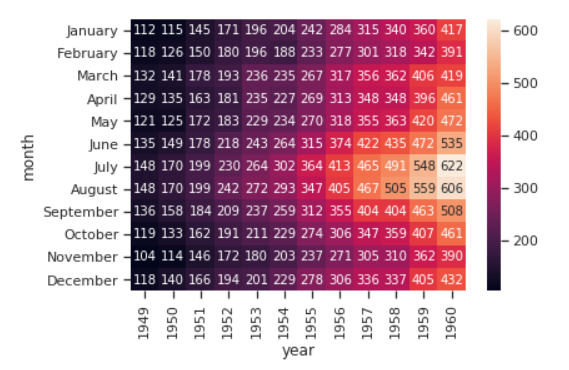### 边框加空格

``````ax = sns.heatmap(flights, linewidths=.5))
``````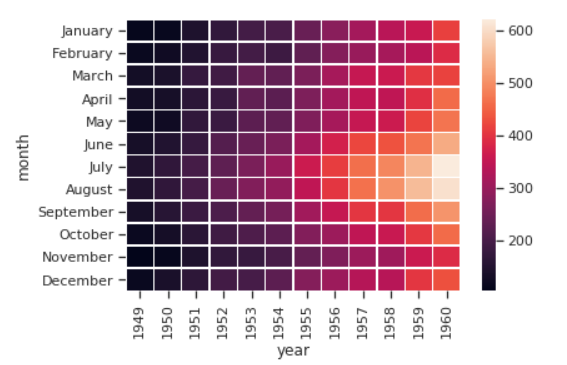### 改变颜色

``````ax = sns.heatmap(flights, cmap="YlGnBu")
``````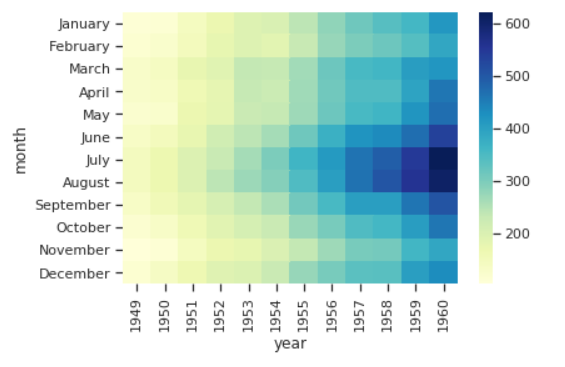### 不显示colorbar

``````ax = sns.heatmap(flights, cbar=False)
``````